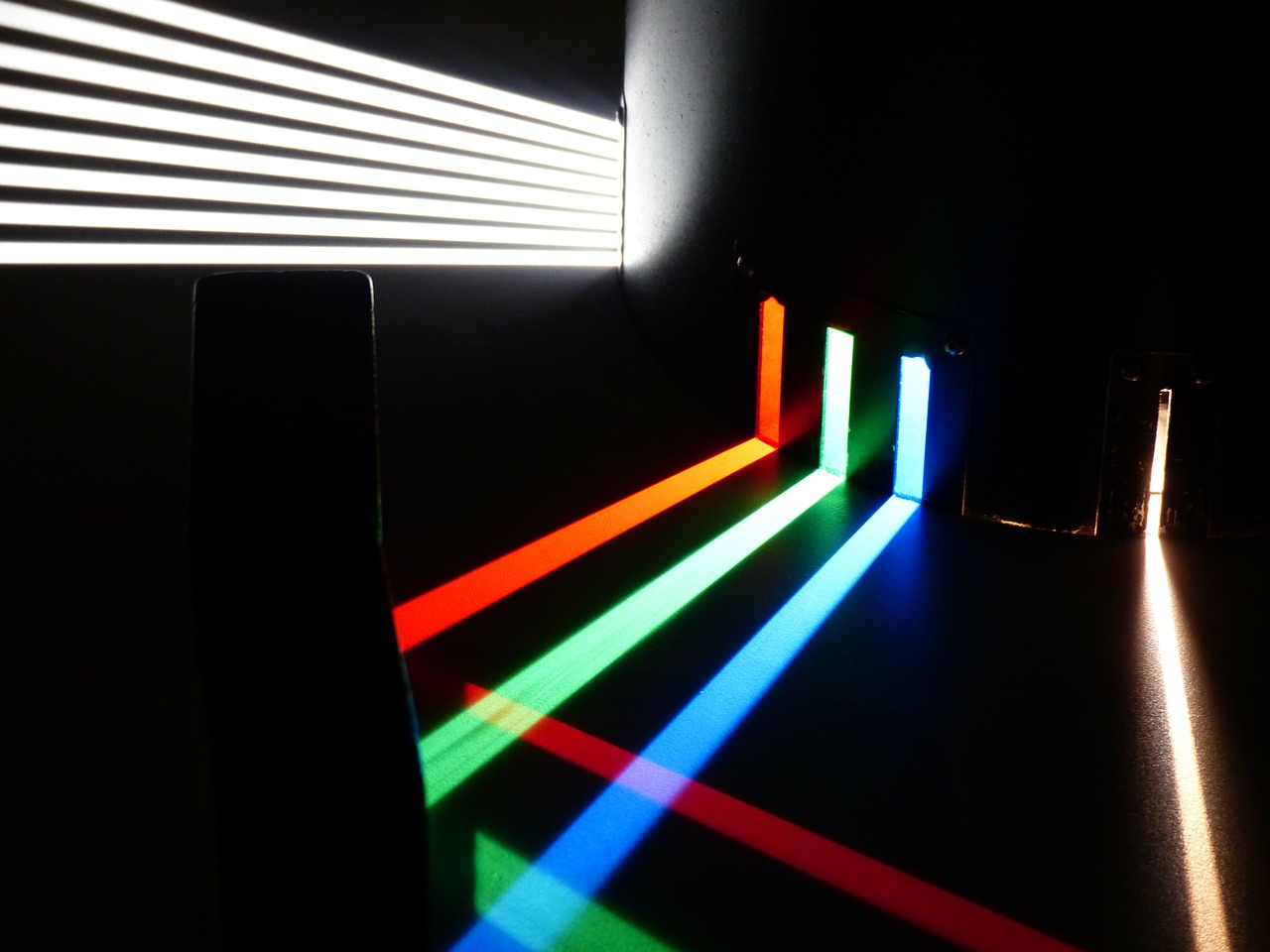# Optics Formula

Optics is an important part of physics. It used in widely in everyday applications like making spectacles to correct vision. So it is important to study it. Let us look into some important optics formula.

## History of Optics

Ancient Indians, Egyptians, Greeks and Romans had all developed theories on optics. Lenses were also made by them. During the medieval ages the Arabians, English were pioneers in developing theories on the speed of light and its study. During the 16th and 17th century, Huygens, Newton developed their own theories on the nature of light its behaviour and contributed to the growth of the field. Eventually, the study of light and measurement of its speed played an important role in dispelling false theories like the presence of ether etc. Quantum physics and can find its origins in the study of optics.## Important Optics Formula

Sin i / Sin r = μ [refractive index of the second medium with respect to the first medium]

Also μ = c/v or v1/v2

μ = Real depth / Apparent depth

Light enters medium a, crosses medium b and then leaves from medium c, then aμc = aμb x bμc

#### Conditions for total internal reflection:

The light should travel from denser to rarer medium.

Angle i > angle ic where ic is the critical angle.

### Refraction at a spherical refracting surface

#### Rarer to a denser medium

1/u + μ2/v = (μ2 – μ1)/R

where μ1 and μ2 are refractive indices of rarer and denser mediums respectively

R is the radius of curvature of the spherical surface.

#### Denser to rarer medium

2/u + μ1/v = (μ1 – μ2)/R

### Lens Maker’s Formula

1/f = (μ – 1)(1/R1 – 1/R2)

Where μ is the refractive index of the material of the lens

R1 and R2 are the radii of curvature of the two surfaces of the lens.

Lens formula: 1/v – 1/u = 1/f

Linear magnification: m = hi/ho = v/u

Power of a lens: P = 1/f if f is in meters Units of P: Dioptre D

### Combination of two thin lenses

#### Lenses in contact

1/F = 1/f1 + 1/f2 => P = P1 + P2 and m = m1 x m2

#### Lenses separated by a finite distance

1/F = 1/f1 + 1/f2 – d/f1f2

### Magnifying power

#### Simple microscope

m = 1 + D/f where D = least distance of distinct vision = 2.5 cm

#### Compound microscope

The ratio of the angle subtended at the eye by the final image to the angle subtended at the eye by the object where both the final image and object are situated at the least distance of distinct vision.

m = L/fo[1 + (D/fe)]

L is the length of the microscope tube

fo is the focal length of the objective

fe is the focal length of the eyepiece

### Resolving power of a microscope

Resolving power = 1/d = (2μSinθ)/λ

where μ is the refractive index of the medium

λ is the wavelength of light

θ is half-angle of the cone of light from the point object to the objective lens

### Resolving power of a telescope

Resolving power = 1/dθ = D/1.22λ

where D is the diameter of the object lens

λ is the wavelength of light

### Laws of reflection

Angle i = angle r

The incident ray, reflected ray and the normal at the point of incidence all lie in the same plane

1/v + 1/u = 1/f

m = hi/ho= -v/u

## Solved Examples for Optics Formula

Q: A small telescope has a lens with the focal length of 180 cms. And the eyepiece has the focal length of 9cm. Find the magnifying power of this telescope by using the optics formula.
Solution: Given parameters are,
The focal length of lens $$f_0$$ = 180
The focal length of eyepiece $$f-1$$ = 9cms
Magnifying power formula = $$\frac {f_0}{f_1}$$ = $$\frac {180}{9}$$ = 20
Share with friends

## Customize your course in 30 seconds

##### Which class are you in?
5th
6th
7th
8th
9th
10th
11th
12th
Get ready for all-new Live Classes!
Now learn Live with India's best teachers. Join courses with the best schedule and enjoy fun and interactive classes.Ashhar Firdausi
IIT Roorkee
BiologyDr. Nazma Shaik
VTU
ChemistryGaurav Tiwari
APJAKTU
Physics
Get Started

## Browse

##### Physics Formulas7 Followers

Most reacted comment
5 Comment authorsRecent comment authors
Subscribe
Notify ofGuest

Typo Error>
Speed of Light, C = 299,792,458 m/s in vacuum
So U s/b C = 3 x 10^8 m/s
Not that C = 3 x 108 m/s
to imply C = 324 m/s
A bullet is faster than 324m/sGuest

I have realy intrested to to this topicGuest
umer

m=f/a correct thisGuest
B. Akshaya

M=f/gGuest

Interesting studiesGuest
Yashdeep tiwari

It is already correct f= ma by second newton formula…

## Question Mark?

Have a doubt at 3 am? Our experts are available 24x7. Connect with a tutor instantly and get your concepts cleared in less than 3 steps.